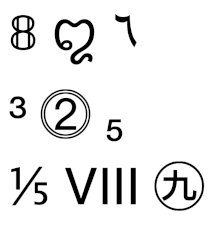# Unicode numbers

There are 10 digits in ASCII, and I bet you can guess what they are. In ASCII, a digit is a decimal is a number.

Things are much wilder in Unicode. There are hundreds of decimals, digits, and numeric characters, and they’re different sets.The following Python code loops through all possible Unicode characters, extracting the set of decimals, digits, and numbers.

```    numbers  = set()
decimals = set()
digits   = set()

for i in range(1, 0x110000):
ch = chr(i)
if ch.isdigit():
if ch.isdecimal():
if ch.isnumeric():
```

These sets are larger than you may expect. The code

`    print(len(decimals), len(digits), len(numbers))`

tells us that the size of the three sets are 650, 778, and 1862 respectively.

The following code verifies that decimals are a proper subset of digits and that digits are a proper subset of numerical characters.

`    assert(decimals < digits < numbers)`

Now let’s look at the characters in the image above. The following code describes what each character is and how it is classified. The first three characters are digits, the next three are decimals but not digits, and the last three are numeric but not decimals.

```    from unicodedata import name
for c in "꩓٦":
print(name(c))
assert(c.isdecimal())
for c in "³⓶₅":
print(name(c))
assert(c.isdigit() and not c.isdecimal())
for c in "⅕Ⅷ㊈":
print(name(c))
assert(c.isnumeric() and not c.isdigit())
```

The names of the characters are

1. MATHEMATICAL DOUBLE-STRUCK DIGIT EIGHT
2. CHAM DIGIT THREE
3. ARABIC-INDIC DIGIT SIX
4. SUPERSCRIPT THREE
5. DOUBLE CIRCLED DIGIT TWO
6. SUBSCRIPT FIVE
7. VULGAR FRACTION ONE FIFTH
8. ROMAN NUMERAL EIGHT
9. CIRCLED IDEOGRAPH NINE

Update: See the next post on ideographic numerals.

Update: There are 142 distinct numbers that correspond to the numerical value associated with a Unicode character. This page gives a list of the values and an example of each value.